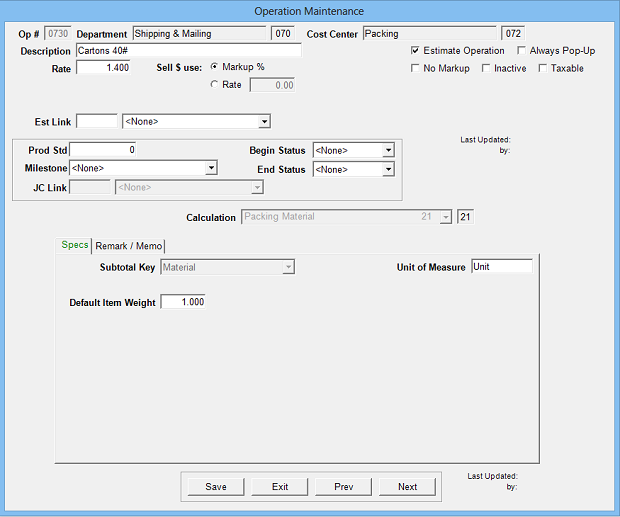Estimates packing material costs. Preceding a Calc 21 operation with a Calc 20 operation will automatically calculate the packing units.

Operation Setup Requirements:
1. Description
2. Rate: Cost per unit (carton, skid, etc.)
3. Sell \$ use: Markup % (recommended) or alternate sell rate
4. Calc: 21
5. Sub Key: Material
6. Default Item Weight: Pounds per unit (empty weight of carton, etc.)Estimate Specification Input Prompts:

1. Units: Number of packages (if Calc 20 precedes this operation, matching units will default)
2. Unit Weight: Pounds per unit (only used to calculate total form ship pounds)
3. Markup %
4. Remarks
5. MemoCalculation:

Units x Rate = Cost \$

Cost \$ x Markup % = Markup \$

Cost \$ + Markup \$ = Sell \$